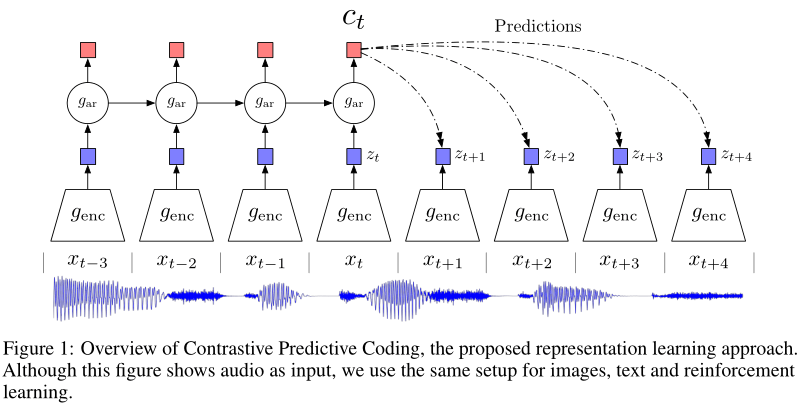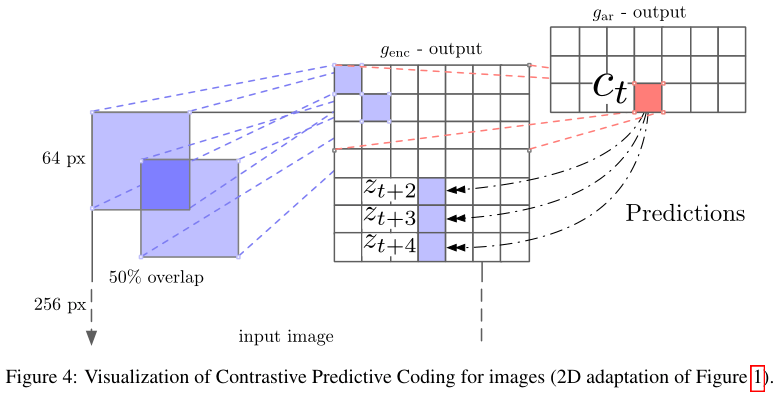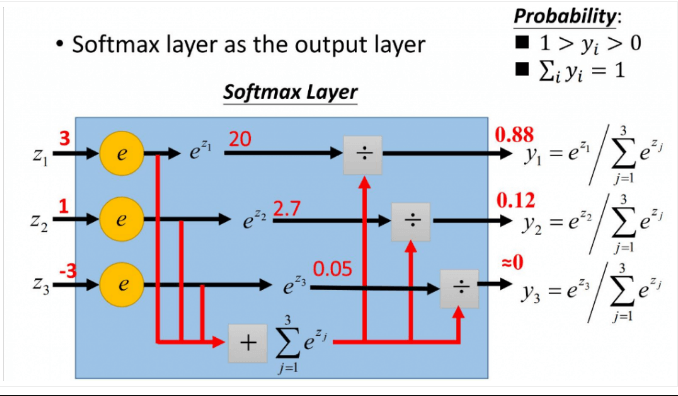## 1 Introduce

• 如何更好的从原始观测值中建模high-level representarion
• 理想情况下的学习的representation到底是什么样的？是否可以使用无监督学习进行理想的representation learning？
• 作者将无监督学习与预测编码的思想相结合，提出了是否学习到一些能对未来有很好预测效果的representation？

• 将高维数据压缩到更紧凑的隐空间中，在其中条件预测更容易建模。
• 我们使用强大的自回归模型在这个隐空间中预测未来的许多步骤。
• 依靠噪声对比估计（Noise-Contrastive Estimation）$^{}$来计算损失函数（和自然语言模型中的学习单词嵌入方式类似），从而可以对整个模型进行端到端的训练。
• 最终提出的Contrastive Predictive Codeing（CPC）方法可以用相同的机制在图像、语音、自然语言、强化学习等多个模态的数据上都能学习到高级信息。

## 2 Motivation && Intuitions

$$I(x ; c)=\sum_{x, c} p(x, c) \log \frac{p(x \mid c)}{p(x)} \tag{1}$$

\begin{aligned} I(x;c) &=\sum_{x, c} p(x, c) \log \frac{p(x \mid c)}{p(x)} \\ &=\sum_{x, c} p(x, c) \log p(x \mid c)-\sum_{x, c} p(x, c) \log p(x) \\ &=\sum_{c} p(c) \sum_{x} p(x \mid c) \log p(x \mid c)-\sum_{x} \sum_{c} p(x, c) \log p(x) \\ &=-H(x \mid c)+H(x) \\ &=H(x)-H(x \mid c) \end{aligned}

$$H(x)=-\sum_x p(x)logp(x)$$

## 3 Method

### 3.1 Contrastive Predicting Coding

understanding-of-CPC$$f_{k}\left(x_{t+k}, c_{t}\right) \propto \frac{p\left(x_{t+k} \mid c_{t}\right)}{p\left(x_{t+k}\right)} \tag {2}$$

$$f_{k}\left(x_{t+k}, c_{t}\right)=\exp \left(z_{t+k}^{T} W_{k} c_{t}\right) \tag{3}$$

### 3.2 InfoNCE Loss

$$\mathcal{L}_{\mathrm{N}}=-\underset{X}{\mathbb{E}}\left[\log \frac{f_{k}\left(x_{t+k}, c_{t}\right)}{\sum_{x_{j} \in X} f_{k}\left(x_{j}, c_{t}\right)}\right] \tag{4}$$ 其中 $X={\{x_1,x_2,\dots,x_N\}}$ 是一组样本，这里面 $(x_{t+k},c_t$) 可以看成正样本对，$(x_j,c_t)$可以看成负样本对。**因此为了优化该损失，我们希望分子尽可能大，分母尽可能小。这也就符合我们对于 $x$ 和 $c$ 互信息的要求，即正样本对之间的互信息更大，负样本对之间的互信息更小。优化该损失，实际上就是最大化$x_{t+k}$ 和 $c_t$ 间的互信息。**

\begin{aligned} p\left(d=i \mid X, c_{t}\right) &=\frac{p\left(x_{i} \mid c_{t}\right) \prod_{l \neq i} p\left(x_{l}\right)}{\sum_{j=1}^{N} p\left(x_{j} \mid c_{t}\right) \prod_{l \neq j} p\left(x_{l}\right)} \ &=\frac{\frac{p\left(x_{i} \mid c_{t}\right)}{p\left(x_{i}\right)}}{\sum_{j=1}^{N} \frac{p\left(x_{j} \mid c_{t}\right)}{p\left(x_{j}\right)}} \end{aligned} \tag{5}

### 3.3 Estimating the MI with InfoNCE

\begin{aligned} \mathcal{L}_{\mathrm{N}}^{\mathrm{opt}} &=-\underset{X}{\mathbb{E}} \log \left[\frac{\frac{p\left(x_{t+k} \mid c_{t}\right)}{p\left(x_{t+k}\right)}}{\frac{p\left(x_{t+k} \mid c_{t}\right)}{p\left(x_{t+k}\right)}+\sum_{x_{j} \in X_{\mathrm{ncg}}} \frac{p\left(x_{j} \mid c_{t}\right)}{p\left(x_{j}\right)}}\right] \\ &=\underset{X}{\mathbb{E}} \log \left[1+\frac{p\left(x_{t+k}\right)}{p\left(x_{t+k} \mid c_{t}\right)} \sum_{x_{j} \in X_{\text {neg }}} \frac{p\left(x_{j} \mid c_{t}\right)}{p\left(x_{j}\right)}\right] \\ & \approx \underset{X}{\mathbb{E}} \log \left[1+\frac{p\left(x_{t+k}\right)}{p\left(x_{t+k} \mid c_{t}\right)}(N-1) \underset{x_{j}}{\mathbb{E}} \frac{p\left(x_{j} \mid c_{t}\right)}{p\left(x_{j}\right)}\right] \\ &=\underset{X}{\mathbb{E}} \log \left[1+\frac{p\left(x_{t+k}\right)}{p\left(x_{t+k} \mid c_{t}\right)}(N-1)\right] \\ & \geq \underset{X}{\mathbb{E}} \log \left[\frac{p\left(x_{t+k}\right)}{p\left(x_{t+k} \mid c_{t}\right)} N\right] \\ &=-I\left(x_{t+k}, c_{t}\right)+\log (N) \end{aligned}

$$I\left(x_{t+k}, c_{t}\right)\geq \log (N)-L_N^{opt}$$

InfoNCE也与MINE有关$^{}$，不失一般性的定义 $f(x,c)=e^{F(x,c)}$：

\begin{aligned} \underset{X}{\mathbb{E}}\left[\log \frac{f(x, c)}{\sum_{x_{j} \in X} f\left(x_{j}, c\right)}\right]&=\underset{(x, c)}{\mathbb{E}}[F(x, c)]-\underset{(x, c)}{\mathbb{E}}\left[\log \sum_{x_{j} \in X} e^{F\left(x_{j}, c\right)}\right] \\ &= \underset{(x, c)}{\mathbb{E}}[F(x, c)]-\underset{(x, c)}{\mathbb{E}}\left[\log \left(e^{F(x, c)}+\sum_{x_{j} \in X_{\text {neg }}} e^{F\left(x_{j}, c\right)}\right)\right] \\ & \leq \underset{(x, c)}{\mathbb{E}}[F(x, c)]-\underset{c}{\mathbb{E}}\left[\log \sum_{x_{j} \in X_{\mathrm{neg}}} e^{F\left(x_{j}, c\right)}\right] \\ & =\underset{(x, c)}{\mathbb{E}}[F(x, c)]-\underset{c}{\mathbb{E}}\left[\log \frac{1}{N-1} \sum_{x_{j} \in X_{\text {neg }}} e^{F\left(x_{j}, c\right)}+\log (N-1)\right] \end{aligned}

$$I \widehat{(X ; Z)}_{n}=\sup _{\theta \in \Theta} \mathbb{E}_{\mathbb{P}_{X Z}^{(n)}}\left[T_{\theta}\right]-\log \left(\mathbb{E}_{\mathbb{P}_{X}^{(n)} \otimes \hat{\mathbb{P}}_{Z}^{(n)}}\left[e^{T_{\theta}}\right]\right)$$ 最终推导的结果几乎等价于MINE估计器，只是多了一个常数 $\underset{c}{\mathbb{E}}[\log (N-1)]$ 。作者声明，当面临困难任务时，InfoNCE与MINE表现都很好，而如果目标很容易根据上下文进行预测的简答任务，则MINE会变得不稳定。

## 4 ExperimentsCPC在计算机视觉领域的用法

### 相关知识补充：

（1）表征学习（Representation Learning）：学习数据的表征，以便在构建分类器或其他预测器时更容易提取有用的信息，无监督学习也属于表征学习。

（2）自回归模型（Autoregressive Model，AR模型）：是统计上一种处理时间序列的方法，用同一变数，例如$x$的之前各期，即$x_1$至$x_{t-1}$来预测本期$x_t$的表现，并假设它们为线性关系。这是从回归分析的线性回归发展而来，只不过不是$x$预测$y$，而是用$x$预测$x$（自己），所以叫做自回归。

$$X_{t}=c+\sum_{i=1}^{p} \varphi_{i} X_{t-i}+\varepsilon_{t}$$

（3）隐空间（latent space）：GAN的输入空间，指隐变量$z$的样本空间，”隐变量”可以理解为控制数据$X$生成的”幕后之手“。在统计机器学习中，隐变量生成式模型“生成”数据$X$的背后逻辑是，通过建模联合分布$p_θ(z,X)$ ，再从中采样得到 $(z,X)$ 对。具体操作是，为隐变量选择一个容易采样的分布，如高斯，再通过神经网络建模$p_θ(X|z)$，从而采样得到生成的数据。

（4）互信息（Mutual Information）：指变量间的相关性，通常用$I(X;Y)$表示$X$和$Y$之间的互信息:
$$I(X ; Y)=\sum_{x \in X} \sum_{y \in Y} p(x, y) \log \frac{p(x \mid y)}{p(x)}$$
$I(X;Y)$越大说明两者关系越密切。

$$I(x, c) =\sum_{x, c} p(x, c) \log \frac{p(x \mid c)}{p(x)}$$

\begin{aligned} I(x, c) &=\sum_{x, c} p(x, c) \log \frac{p(x \mid c)}{p(x)} \\ &=\sum_{x, c} p(x, c) \log \frac{p(x \mid c) p(c)}{p(x) p(c)} \\ &=\sum_{x, c} p(x, c) \log p(x \mid c)-\sum_{x, c} p(x, c) \log p(x) \\ &=\sum_{c} p(c) \sum_{x} p(x \mid c) \log p(x \mid c)-\sum_{x} \sum_{c} p(x, c) \log p(x) \\ &=H(x)-H(x \mid c) \end{aligned}

$$H(x)=-\sum_x p(x)logp(x)$$

（5）对比损失（Contrastive Loss）

（6）NCE Loss

https://blog.csdn.net/weixin_40901056/article/details/88568344

（7）softmax函数

softmax用于多分类过程中，它将多个神经元的输出，映射到（0,1）区间内，可以看成概率来理解，从而来进行多分类。

$$S_{i}=\frac{e^{i}}{\sum_{j} e^{j}}$$（8）各种熵：

https://zhuanlan.zhihu.com/p/35423404

https://www.jianshu.com/p/2ea0406d0793

https://zhuanlan.zhihu.com/p/129076690

https://zhuanlan.zhihu.com/p/75517749

https://zhuanlan.zhihu.com/p/137076811

 Michael Gutmann and Aapo Hyvärinen. Noise-contrastive estimation: A new estimation principle for unnormalized statistical models. In Proceedings of the Thirteenth International Conference on Artificial Intelligence and Statistics, pages 297–304, 2010.

 Laurenz Wiskott and Terrence J Sejnowski. Slow feature analysis: Unsupervised learning of invariances. Neural computation, 14(4):715–770, 2002.

 Ishmael Belghazi, Sai Rajeswar, AristideN Baratin, R Devon Hjelm, and Aaron Courville. Mine: Mutual information neural estimation. 2018.